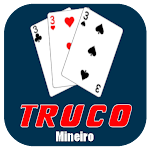ID: br.com.msivtronic.trucomineiro

• Author:

• Version:

Varies with device

• Update on:

## The description of Truco - mineiro version

THE GAME
Truco is a card game that is played in pairs and uses a deck with forty cards (without 8, 9, 10 cards).
The matches are called 'hands' and each hands has three rounds.
One hand can value two, four, six, ten or twelve points.

HOW TO PLAY
The player that plays the highest card wins the round and the pair
that wins more rounds wins the hand.
In each hand there are two types of cards: commons and trumps cards.
1) Rank of common cards
From high to low: 3, 2, A, K, J, Q, 7, 6, 5, 4(not depend of suit)

2) Rank of trumps cards
The rank of trumps cards depend on the suit
From high to low: Four of clubs(Zap), Seven of hearts(Copeta), Ace of spades(Espadilha) and Seven of diamonds(Ouros)

3) Increasing the value of hand
3.1)Requesting truco
All players, in your turn, can request 'Truco' and the opponent pair must answer if they accept, run or ask six.
One pair can't request 'Truco' twice in a row.

If the pair accepts the request of truco, the value of hand will be four.
If the pair doesn't accept (run) the request of truco, the opponent pair will earn two points.
If the pair askes six, the opponent pair must answer if they accept, run or ask ten.

If the pair accepts the asked of six , the value of hand will be six.
If the pair doesn't accept the asked of six , the opponent pair will earn four points.
If the pair askes ten, the opponent pair must answer if they accept, run or ask twelve.

If the pair accepts the asked of ten, the value of hand will be ten.
If the pair doesn't accept the asked of ten, the opponent pair will earn six points.
If the pair askes twelve, the opponent pair must answer if they accept or run.

If the pair accepts the asked of twelve, the value of hand will be twelve.
If the pair doesn't accept the asked of twelve, the opponent pair earn ten points.

SPECIAL CASES
1) In case of draw
1.1) First round : If the first round finishes drawed the pair that win the second round will win the hand.
1.2) Second round : If the second round finishes drawed the pair that win the
first round will win the hand.
1.3) Third round : If the third round finishes drawed the pair that win the first round will win the hand.
1.4) All rounds : If all rounds finish drawed the pair that deal the cards will win the hand.

2) Hand of ten
The pair that score ten points first can decide that will play or not the next hand and the pair's players can see the cards of each other.
If the pair choose to play, The value of hand will be four points and if the pair
choose not to play, the opponent pair will earn two points.
Nobody can request 'Truco' in Hand of ten.

3) Hand of iron
When both pairs score ten points the next hand will be the 'Hand of Iron' and nobody can request 'truco'.The pair who wins this hand will win the game.

WINNING THE GAME
The pair that score twelve or more points first wins the game.